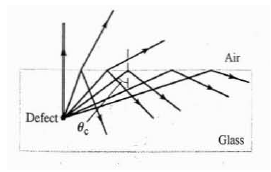# Problem: The figure shows some light rays reflected from a small defect in the glass toward the surface of the glass.(a) If θc = 40.00°, what is the index of refraction of the glass?(b) Is there any point above the glass at which the viewer would not be able to see the defect? Explain.

###### FREE Expert Solution

Critical angle:

$\overline{){{\mathbf{\theta }}}_{{\mathbf{c}}}{\mathbf{=}}{\mathbf{s}}{\mathbf{i}}{{\mathbf{n}}}^{\mathbf{-}\mathbf{1}}{\mathbf{\left(}}\frac{{\mathbf{\eta }}_{\mathbf{1}}}{{\mathbf{\eta }}_{\mathbf{2}}}{\mathbf{\right)}}}$

(a)

Let the refractive index of air be η1 and the refractive index of the glass be η2

We know that the refractive index of air, η1 = ηa = 1

96% (308 ratings)###### Problem Details

The figure shows some light rays reflected from a small defect in the glass toward the surface of the glass.(a) If θc = 40.00°, what is the index of refraction of the glass?

(b) Is there any point above the glass at which the viewer would not be able to see the defect? Explain.